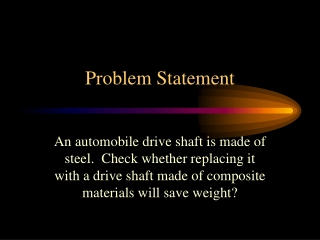DownloadDownload PresentationProblem Statement

# Problem Statement

Télécharger la présentation## Problem Statement

- - - - - - - - - - - - - - - - - - - - - - - - - - - E N D - - - - - - - - - - - - - - - - - - - - - - - - - - -
##### Presentation Transcript

1. Problem Statement An automobile drive shaft is made of steel. Check whether replacing it with a drive shaft made of composite materials will save weight?

2. Design of a Composite Drive ShaftAutar K. Kaw WELCOME TO COMPOSITE MATERIALS

3. Problem Description Following are fixed 1. Maximum horsepower=175 HP @4200rpm 2. Maximum torque = 265 lb-ft @2800rpm 3. Factor of safety = 3 3. Outside radius = 1.75 in 4. Length = 43.5 in

4. Torque in Drive Shaft In first gear, the speed is 2800 rpm (46.67 rev/s) for a ground speed of 23 mph (405 in/sec) Diameter of tire = 27 in Revolutions of tire = (405)/(*27) = 4.78 rev/s Differential ratio = 3.42 Drive shaft speed = 4.78 x 3.42 = 16.35 rev/s Torque in drive shaft = 265 *46.67/16.35 = 755 lb-ft

5. Maximum Frequency of Shaft Maximum Speed = 100 mph Drive Shaft Revs = 16.35 rev/s at 23 mph So maximum frequency = 16.35 *100/23 = 71.1 rev/s (Hz)

6. Design Parameters • Torque Resistance. • Should carry load without failure • Not rotate close to natural frequency. • Need high natural frequency otherwise whirling may take place • Buckling Resistance. • May buckle before failing

7. Steel Shaft - Torque Resistance max / FS= Tc /J Shear Strength max = 25 Ksi Torque T = 755 lb-ft Factor of Safety FS = 3 Outer Radius c = 1.75 in Polar moment of area J = /2 *(1.754 - cin4) Hence, cin =1.69 in, that is, Thickness, t = 1.75-1.69 = 0.06 in = 1/16 in

8. Steel Shaft - Natural Frequency fn = /2 *sqrt( g E I /[ W L4]) g=32.2 ft/s2 E = 30 Msi W = 0.19011 lb/in L = 43.5 in Hence fn = 204 Hz (meets minimum of 71.1Hz)

9. Steel Shaft - Torsional Buckling T = 0.272 *2  r2 t E (t/r)^3/2 r = 1.6875 in t = 1/16 in E = 30 Msi Critical Torsional Buckling Load T = 5519 lb-ft (meets minimum of 755 lb-ft)

10. Designing with a composite

11. Load calculations for PROMAL Nxy = T /(2  r 2) T = 755 lb-ft r = 1.75 in Nxy = 470.8 lb / in

12. Composite Shaft - Torque Resistance Inputs to PROMAL: Glass/Epoxy from Table 2.1 Lamina Thickness = 0.0049213 in Stacking Sequence: (45/-45/45/-45/45)s Load Nxy = 470.8 lb / in Outputs of PROMAL: Smallest Strength Ratio = 4.1 Thickness of Laminate: h = 0.0049213*10 = 0.04921 in

13. Composite Shaft - Natural Frequency fn = /2 * sqrt( g Ex I /[ W L4]) g = 32.2 ft/s2 Ex = 1.821 Msi I = 0.7942 in4 W = 0.03999 lb/in (Specific gravity = 2.076) L = 43.5 in Hence fn = 98.1 Hz (meets minimum 71.1 Hz)

14. Composite Shaft - Torsional Buckling T = 0.272 *2  rm2 t (Ex Ey3)1/4 (t/rm)3/2 rm = 1.75 - 0.04921/2 = 1.72539 in t = 0.04921 in Ex = 1.821 Msi Ey = 1.821 Msi T = 183 lb-ft (does not meet 755 lb-ft torque)

15. Comparison of Mass